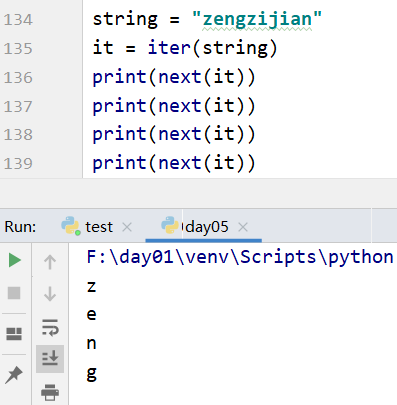# 暑期实训第五天课---迭代器、生成器

map : 按指定规则对序列进行映射。参数一：表示规则的函数 参数二，参数三：表示要被映射的序列
filter：按指定规则对序列中的元素进行过滤
reduce :指按指定的规则对序列中的元素进行累计运算

Zip的使用，将两个列表，按照索引的顺序组合起来

word1 = words[:-1] #将words里单词从第一个到倒数第二个，不包含最后一个
word2 = words[1:] #第二个到最后一个单词
for word in zip(word1,word2):

def mimic_dict(file_name):
'''
1.读取文件内容
2.把文件内容中的字符串按空格进行切分
3.统计每个单词后面紧跟单词列表
:param file_name:
:return:
'''
with open(file_name)as file:

content = content.replace('.\n'," ")
words = content.split(" ")

word1 = words[:-1]

word2 = words[1:]

word_context_dict = {}

#zip函数作用是组合两个列表，上下一一对应
for word in zip(word1,word2):

#word 是一个元组，第一个元素是word1里的元素，第二个元素是第一个元素紧跟的单词
if word in word_context_dict.keys():

word_context_dict[word].append(word)
else:
word_context_dict[word] = [word]

return word_context_dict

print(mimic_dict("zen.txt"))


## 迭代器string = "zengzijian"
it = iter(string)
for each in string:
print(each)


while True:
try:
each = next(it)
except StopIteration:
break
print(each)


z
e
n
g
z
i
j
i
a
n


iter 和 next 都对应着魔法变量：

__iter__   和 __next__


self.a,self.b = self.b,self.a+self.b


class fibs:
def __init__(self):
self.a = 0
self.b = 1
def __iter__(self):
return self
def __next__(self):
self.a,self.b = self.b,self.a+self.b
return self.a


l = fibs()
for each in l :
if each < 100:
print(each)
else:
break


## 生成器 yield

def libs():
a = 0
b = 1
while True:
a,b = b,a+b
yield a

for each in libs():
if each < 100:
print (each,end=" ")
else:
break


1 1 2 3 5 8 13 21 34 55 89

02-2330
11-2439803-12548
08-0571
11-022023
06-10231
06-262415
03-022479
04-03247